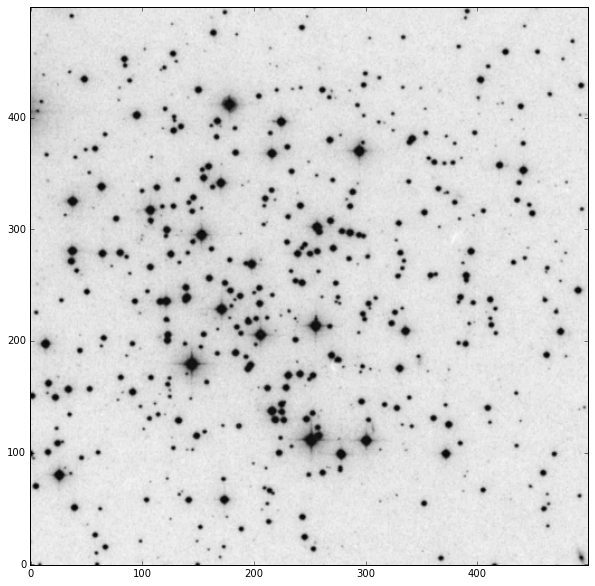# Affiliated Packages: photutils¶

## Source detection and aperture photometry¶

The following example uses photutils to find sources in an astronomical image and perform circular aperture photometry on them. This example requires the following packages:

### Installation¶

You can install the required package with either conda or pip:

• conda -c astropy install photutils

• pip install photutils

### Requirements¶

• astropy v1.0 or later
• photutils v0.1 or later
• numpy 1.6 or later
• scipy
• scikit-image
• matplotlib

We start by loading an image from the bundled datasets and selecting a subset of the image. We then subtract a rough estimate of the background, calculated using the image median. In the remainder of this example, we assume that the data is background-subtracted.

In :
import numpy as np
from photutils import datasets

image = hdu.data[300:800, 300:800].astype(float)
image -= np.median(image)

In :
import matplotlib.pylab as plt
%matplotlib inline

plt.rc('figure', figsize=(10, 10))
plt.imshow(image, cmap='gray_r', origin='lower')

Out:
<matplotlib.image.AxesImage at 0x109db3b70>## Source Detection¶

Photutils supports several source detection algorithms. For this example, we use photutils.daofind to detect the stars in the image. We set the detection threshold at the 3-sigma noise level, estimated using the median absolution deviation of the image. The parameters of the detected sources are returned as an Astropy Table.

In :
from photutils import daofind

sources = daofind(image, fwhm=4., threshold=3.*bkg_sigma)
print(sources)

 id   xcentroid     ycentroid    ...  peak       flux           mag
--- ------------- -------------- ... ------ ------------- ----------------
1 8.32505593822 0.361671501059 ... 7816.0 7.08850256949   -2.12638625281
2 59.5284509248 0.577005398656 ... 7583.0 9.55738167619   -2.45084732501
3 149.486612917  0.49229213635 ... 6874.0 8.48761294391   -2.32196391611
4  99.785726065  3.67338836764 ...  545.0 1.01269842928 -0.0137003409498
5 114.001684611  6.14804716094 ... 2587.0 2.42418756125  -0.961415546227
6  367.13921519  5.61787322663 ... 9030.0 7.44421807403   -2.17954771762
7 492.713278555  5.96981121705 ... 8897.0 6.97318814147   -2.10857845733
8 52.5246058408  6.82359774406 ...  777.0 1.16296608804  -0.163917627338
9 169.579793605  6.62873717122 ... 5978.0 6.25142389508    -1.9899473711
10  433.66940802  8.01492850099 ...  879.0 1.04112148702 -0.0437535242212
...           ...            ... ...    ...           ...              ...
752 389.053072435  488.990503105 ... 7863.0 8.57152613383   -2.33264538401
753  247.42790774  490.111412927 ... 4806.0 5.17405407242    -1.7845774077
754 36.7635046156  490.596411009 ... 8709.0 9.07927059509   -2.39512739959
755 173.832155611  493.935871101 ... 8754.0 9.93047446329   -2.49242499734
756  390.53720049  494.918568556 ... 8952.0 8.41425734199   -2.31253947591
757  214.26806771  496.997708833 ... 3366.0 3.42571588205   -1.33687835281
758 24.9610847363   497.91604648 ... 1222.0 1.14418286794  -0.146238601767
759  28.878836943  498.101767333 ... 1131.0 1.02161935244 -0.0232227778377
760  253.97874348  498.283364592 ... 4750.0 5.31483761326   -1.81372499964
761  207.15897271  498.655797674 ... 4659.0 3.66302253017   -1.40959897472
762 452.782924642  498.660873085 ... 5952.0 4.82774235102   -1.70936021108
Length = 762 rows


## Aperture photometry¶

Using the list of source locations (xcentroid and ycentroid), we can compute the sum of the pixel values within our apertures. For this example we choose circular apertures with a radius of 4 pixels. The photutils.aperture_photometry function returns an Astropy Table with the results of the photometry:

In :
from photutils import aperture_photometry, CircularAperture

positions = (sources['xcentroid'], sources['ycentroid'])
apertures = CircularAperture(positions, r=4.)
phot_table = aperture_photometry(image, apertures)
print(phot_table)

 aperture_sum     xcenter       ycenter
pix           pix
-------------- ------------- --------------
34687.9664115 8.32505593822 0.361671501059
69445.406535 59.5284509248 0.577005398656
65453.6846559 149.486612917  0.49229213635
-3016.31423021  99.785726065  3.67338836764
1748.45985935 114.001684611  6.14804716094
171676.20319  367.13921519  5.61787322663
181257.255496 492.713278555  5.96981121705
-5201.4280847 52.5246058408  6.82359774406
38633.8472726 169.579793605  6.62873717122
-7753.31723912  433.66940802  8.01492850099
...           ...            ...
25370.9222451  247.42790774  490.111412927
118689.250305 36.7635046156  490.596411009
117403.494267 173.832155611  493.935871101
149189.346802  390.53720049  494.918568556
11030.9431808  214.26806771  496.997708833
28555.0718082 24.9610847363   497.91604648
24339.4452655  28.878836943  498.101767333
20873.0018562  253.97874348  498.283364592
19561.2046111  207.15897271  498.655797674
39152.1711172 452.782924642  498.660873085
Length = 762 rows


The sum of the pixel values within the apertures are given in the column aperture_sum. We now plot the image and the defined apertures:

In :
import matplotlib.pylab as plt
%matplotlib inline

plt.rc('figure', figsize=(10, 10))
plt.imshow(image, cmap='gray_r', origin='lower')
apertures.plot(color='blue', lw=1.5, alpha=0.5)